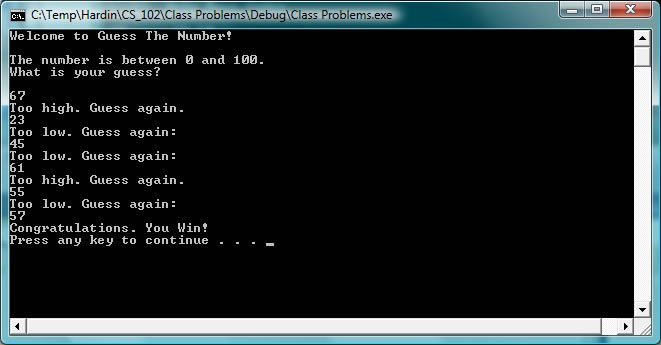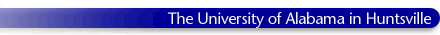#### Class Resources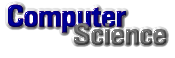# General

##### C Program Template

#include <stdio.h>
#include <stdlib.h>
#include <math.h>

int main(void)

{

printf("\n\n\n");
system("pause");

}

# Chapter 2 Sections 2.1 & 2.2 Screen Output

##### In Class Problem 2: Write a C Program that prints the following to the screen:
 Course: CS 102 Date: August 27, 2012 Author: Sam Jones Problem: In Class Problem 2
##### In Class Problem 3: Write a C program called special.c that prints the special characters backslash, double quote, single quote, and percent sign.

The output should look like this.##### In Class Problem 5: debug_one.c Download the file. Correct the Errors.

Output should look like this.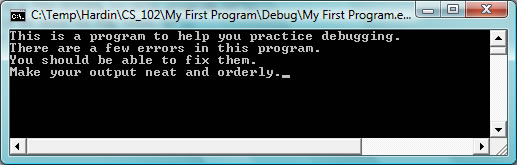# Chapter 2 Sections 2.3, 2.4 & 2.5 Keyboard Input and Calculations

##### In Class Problem 7: Part A: Calculate driving time given distance and avg speed. Part B: Modify program so that it imports values for average speed and distance from keyboard.

Sample output from In Class problems 6 and 7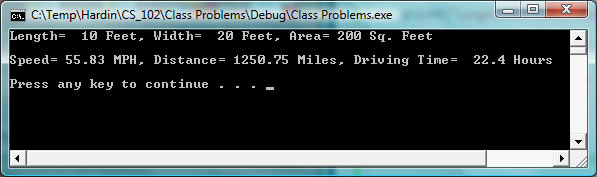##### In Class Problem 8: Temperature ConversionWrite a program that prompts the user to enter a temperature in degrees Celsius and then outputs the equivalent temperature in degees Fahrenheit. F = 9/5 C + 32.0

Sample output for in class problem 8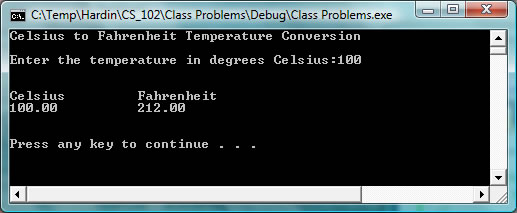##### In Class Problem 9: Body Mass IndexWrite a program that prompts the user to enter their height in feet and inches and their weight in pounds and then computes their Body Mass Index (BMI) where: BMI = weight divided by the height in inches squared and the result multiplied by 703.

Sample output from in class problem 9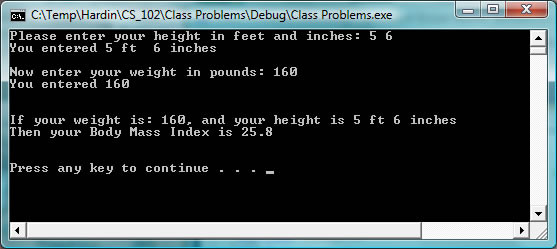##### Volume in cubic feet = 4/3 times PI times the radius cubed. One cubic foot of water contains 7.48 gallons.

Sample output from problem 10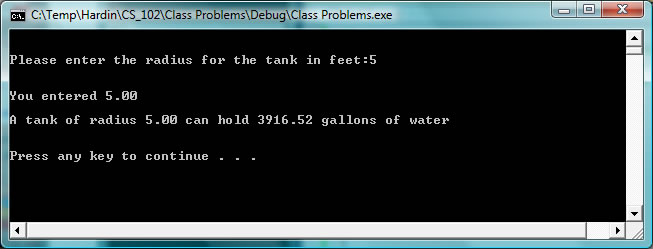# Chapter 2 Section 2.6 Conditionals

##### SOLUTIONS

Solutions to the find largest of three integers problem: To be added
Short solution with two simple if statements: To be added

# Chapter 3

##### Write a program that prompts the user to enter a temperature in degrees Celsius and then outputs the equivalent temperature in degees Fahrenheit.After each value is converted and printed to the screen give the user the option to enter another value. The user will enter 1 to continue and 0 to terminate the program. F = 9/5 C + 32.0

Sample output from problem 13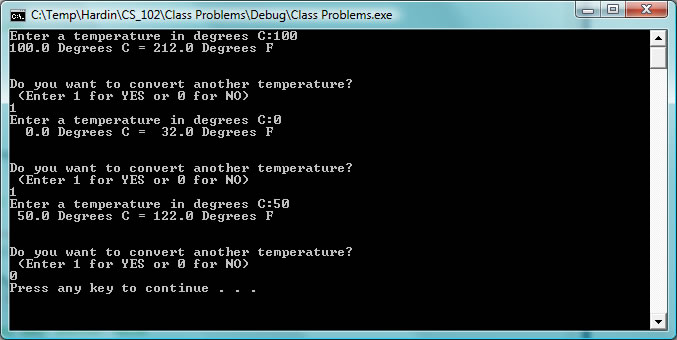##### Design and write a program that prompts the user to enter a starting temperature and an ending temperature in degrees Celsius. The program then creates and prints to the console a table of values for C and F at 5 degree increments.

Part2: Modify the program above to allow the user to input a value for the the increment between values of C in the table.

Sample putput from problem 14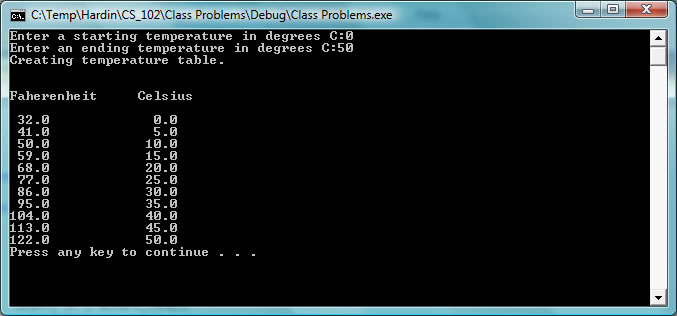##### Design and write a program that allows the user to enter an integer between 1 and 7 that represents the day of the week Sunday through Saturday. After reading the user input the program validates the input. If the value is not valid then the user is given an error message and asked to reenter the value. (Implement this with a While loop) If a valid number is input then the number is tested and the corresponding day is prnted to the console.

Sample output from problem 16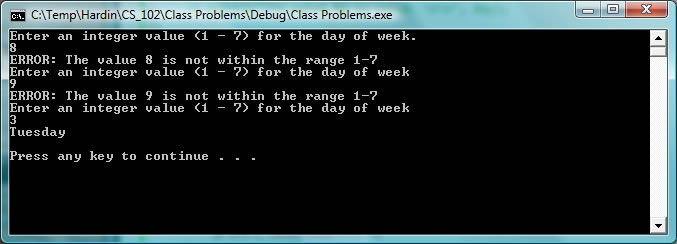##### Part 2: Modify the program to limit the number of guesses to 10. If the user is not able to guess the number in 10 trys print a message to the console that informs them that they have no more guesses and give them the secret number.

Sample execution of the Guess Number program.